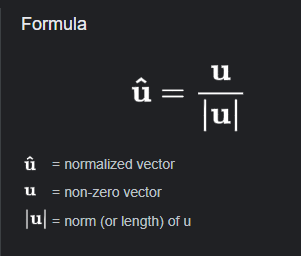# How Does Roblox Calculate LookVectors?

I want to know how Roblox turns any two `Vector3` values into a `LookVector` with values ranging from -1 to 1 via `CFrame.lookAt()`. To make it clearer:

``````...
local Direction = CFrame.lookAt(Part1.Position, Part2.Position)
print(Direction.LookVector) --[[ How does it compute the Vector3s' data and convert it
into a Vector3, specifically with values ranging from -1 to 1?]]
``````

I was assuming that it first gets the direction through subtracting them first, but after some testing, I couldn’t figure out how it was simplified to values ranging from -1 to 1. Also no, this isn’t to fix a problem who’s solution needs this, rather I want to know how Roblox’s function works directly.

Before CFrame.lookAt was released there was CFrame.fromMatrix which is basically the same but showcases the math that needs to be done.

And you are right based on your description of getting the direction.

The range of -1 to 1 is due to the direction vector being unitized such that the length of the vector is equal to one.

1 Like

Thanks, I was thinking about it being some sort of dividing scenario, but it was really just turning the magnitude to 1.

1 Like

You are right again, it is technically just a dividing scenario because that’s how the magnitude is turned to one according to formula for a unit vector.1 Like